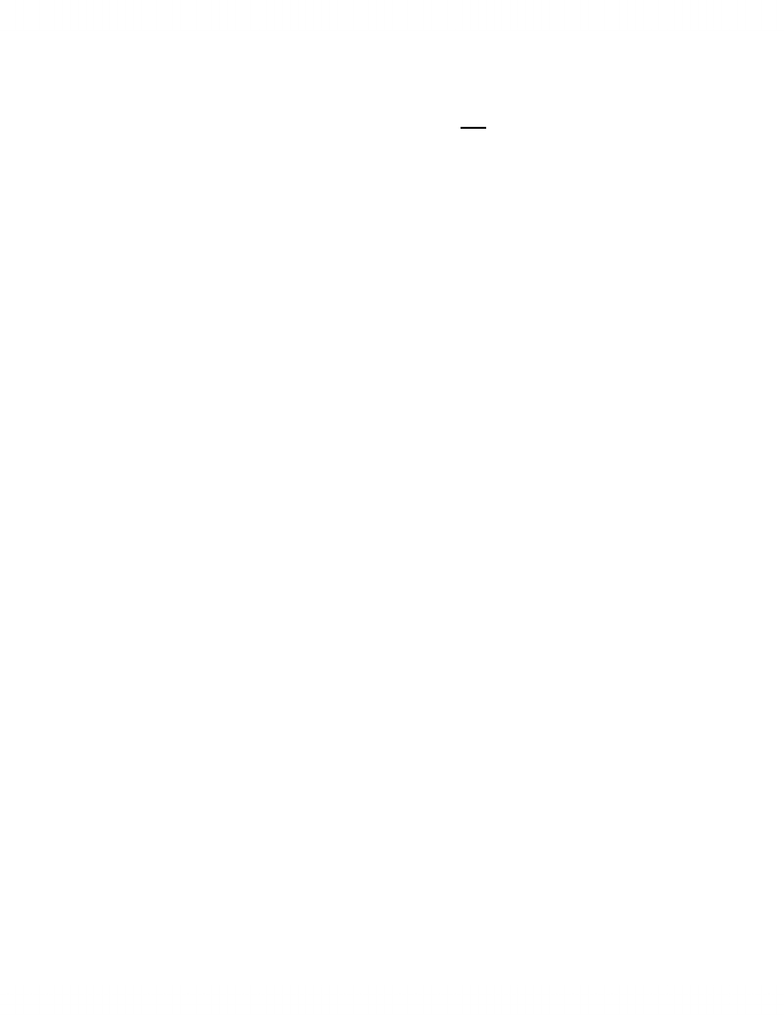# ECON 2X03 Study Guide - Midterm Guide: Isoquant, Pencil, Substitute Good

71 views5 pagesPage 1 of 5
McMaster University Department of Economics
ECON 2G03/2X03
Summer 2011
Test 1
Tuesday May 17 2011
1:30 3:00 (90 minutes) in class
Instructor: H Holmes
MULTIPLE CHOICE
Answer all questions on the scan sheet using HB pencil.
Calculators are permitted.
Hand in the scan and this sheet separately.
TOTAL MC MARKS AVAILABLE: 25
NAME:_______________________________________________________________
STUDENT #:__________________________________________________________
Unlock document

This preview shows pages 1-2 of the document.
Unlock all 5 pages and 3 million more documents.Page 2 of 5
MULTIPLE CHOICE. Choose the one alternative that best completes the statement or
1) A technically efficient technology is one that:
A) maximizes a firm's profits.
B) minimizes the cost of producing a particular level of outputs.
C) maximizes the quantity of output produced from a bundle of inputs.
D) none of the above
2) K and L are perfect substitutes in production in the following production function:
A) min(K,L). B) K + L.
C) KL/2. D) max(K,L).
3) Which of the following is a Cobb-Douglas production function?
A) min(K,L) B) KL/2
C) max(K,L) D) K + L
4) The free-disposal assumption implies that:
A) excess use on an input reduces output unless more of others are used.
B) increases in the MP of an input cannot be negative.
C) garbage disposal is costless.
D) total product always increases as quantity of a variable input.
5) In order to maximize output from a technology that uses one variable input, one must employ the input in such a
way that its:
A) MP is equal to its per-unit price.
B) AP is at a maximum.
C) MP is zero.
D) AP is zero.
6) If TC = 7q3 + 3q + 7, then AVC is:
A) 7q2 + 3q + 7/q. B) 7q2 + 3q.
C) 7q2 + 3. D) 7q2 + 3 + 7/q.
7) If TC = 7q3 + 3q + 7, then AFC is:
A) 7. B) 7q3 + 3q.
C) 3q + 7. D) 7/q.
8) If a firm has TC = 7q3 + 8q2 + 4, then SMC is:
A) 7q3 + 8q2. B) 7q3 + 8q2 + 4.
C) 7q2 + 8q + 4. D) 21q2 + 16q.
9) If a firm's Total Cost function is given by TC = 4 + q2, then:
A) FC = 4 and AVC = q.
B) AVC = q and MC = q.
C) AFC = 4/q and MC = 4 + q.
D) ATC = 4 + q and MC = 2q.
Unlock document

This preview shows pages 1-2 of the document.
Unlock all 5 pages and 3 million more documents.